Function Repository Resource:

# SternBrocot

Get the position of a fraction or vice-versa in the Stern–Brocot sequence

Contributed by: Ed Pegg Jr
 ResourceFunction["SternBrocot"][num] returns the fraction at integer position num in the Stern–Brocot sequence. ResourceFunction["SternBrocot"][frac] returns the integer position of the fraction frac in the Stern–Brocot sequence. ResourceFunction["SternBrocot"][numer,denom] returns the integer position of fraction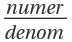in the Stern–Brocot sequence.

## Details

The breadth-first traversal of the Stern–Brocot tree gives the Stern–Brocot sequence, with initial terms as follows: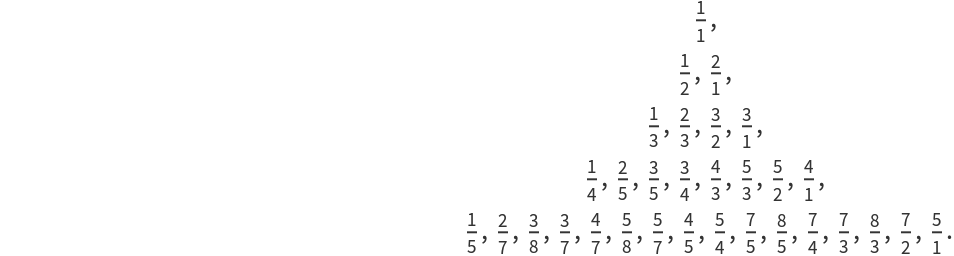In this implementation of ResourceFunction["SternBrocot"], f=0 and f[-n]=-f[n], which may be considered nonstandard.
A bit-reversal permutation of the Stern–Brocot tree gives the Calkin–Wilf tree.
The binary length of the index for fraction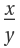equals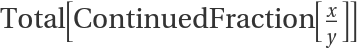. This can lead to unexpected results. For example,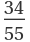has index 341, but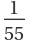has index 18014398509481984.

## Examples

### Basic Examples (2)

Find the position of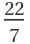in the Stern–Brocot sequence:

 In:=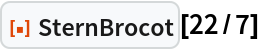Out=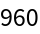Find the position ofin the Stern–Brocot sequence with explicit numerator and denominator:

 In:=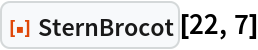Out=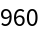Find the fraction at position 960 in the Stern–Brocot sequence:

 In:=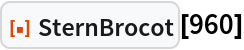Out=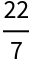Generate terms of the Farey sequence:

 In:=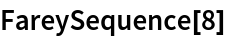Out=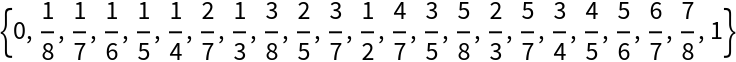Show their positions in the Stern–Brocot sequence:

 In:=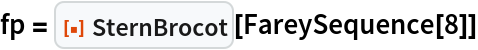Out=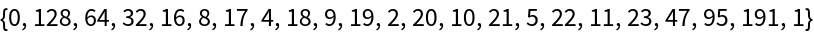Use the positions to generate fractions:

 In:=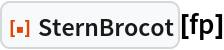Out=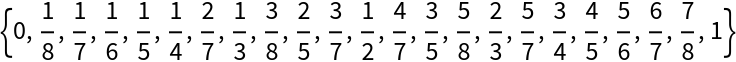### Scope (4)

Show the first 42 terms:

 In:=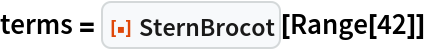Out=Get back the positions using explicit numerators and denominators:

 In:=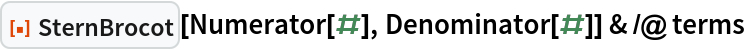Out=Get back the positions by forcing a denominator of 1 everywhere:

 In:=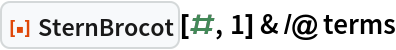Out=A two term form is needed for positions of integers since fractions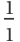,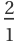,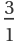,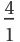are returned as 1, 2, 3, 4:

 In:=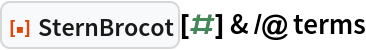Out=### Properties and Relations (6)

Negative values return negative fractions:

 In:=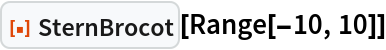Out=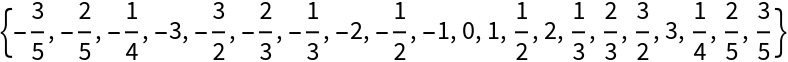Negative fractions correspond to negative positions:

 In:=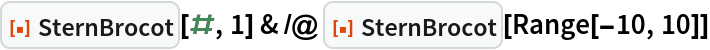Out=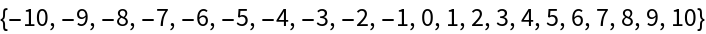Level 5 of the Stern-Brocot tree:

 In:=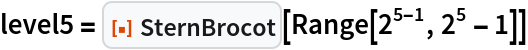Out=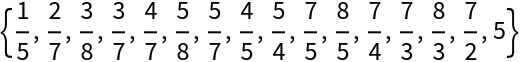Find the Total of the terms of the ContinuedFraction for level 5 of the Stern-Brocot tree:

 In:=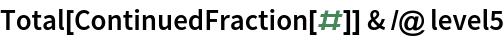Out=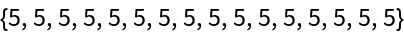For terms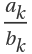on the same level in the Stern–Brocot binary tree,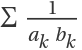=1:

 In:=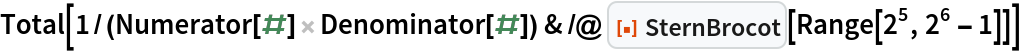Out=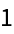The maximal numerator for level n of the Stern-Brocot tree is Fibonacci[n+1]:

 In:=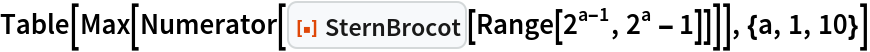Out=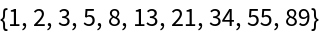An unreduced fraction gives the same value as the reduced fraction:

 In:=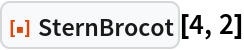Out=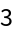In:=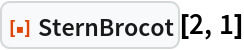Out=Create a plot of the first 256 values:

 In:=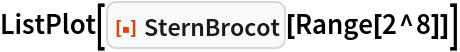Out=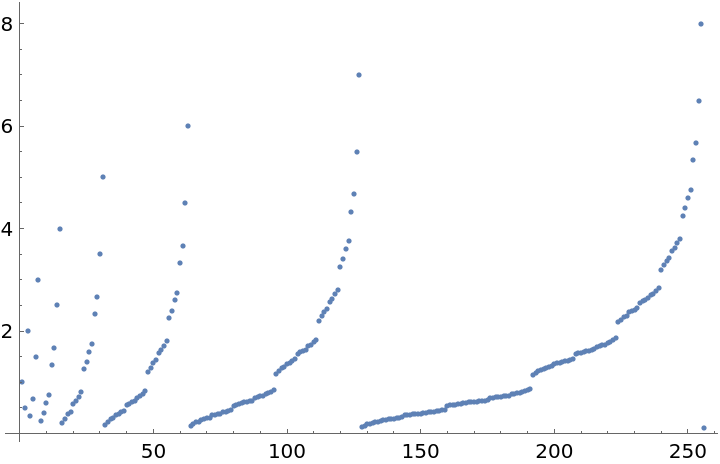Create a plot of indices for the Farey sequence fractions:

 In:=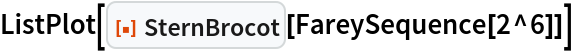Out=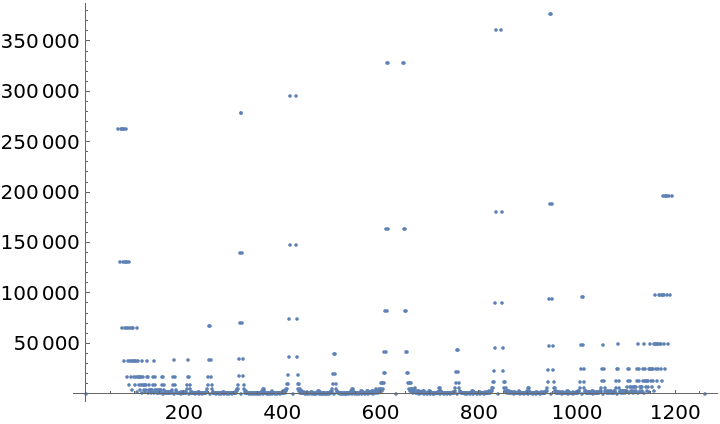### Possible Issues (3)

Fractions at positions 2a-1 are returned as a instead of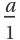:

 In:=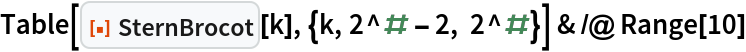Out=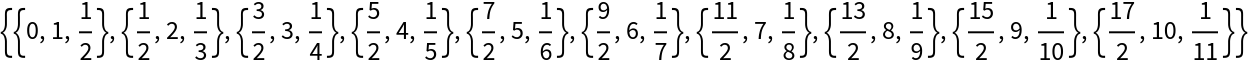SternBrocot returns a Failure if its input is anything other than an integer:

 In:=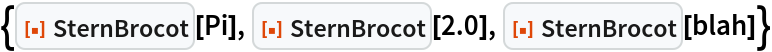Out=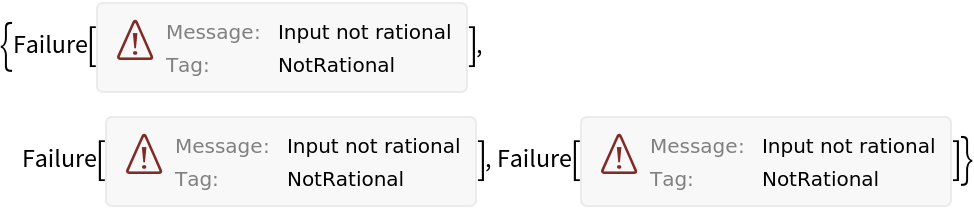Fractions leading to an index with more than a million digits will be flagged as out of range:

 In:=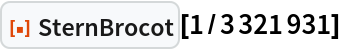Out=Fractions with a million digit index will be evaluated as expected:

 In:=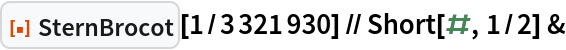Out=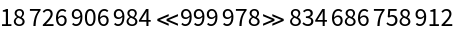### Neat Examples (4)

Find fractions of the order 8 FareySequence missing from the order 5 Stern–Brocot binary tree:

 In:=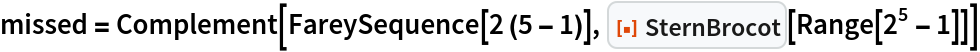Out=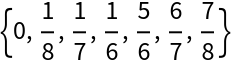Show the Stern–Brocot binary tree:

 In:=Out=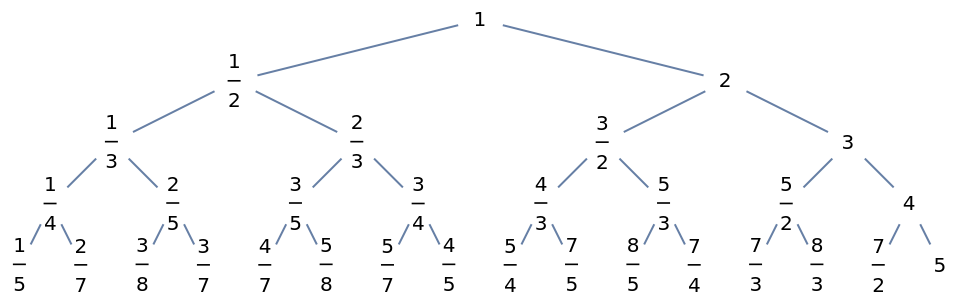Put the Stern–Brocot binary tree into a hydraulic press:

 In:=Out=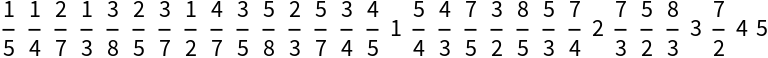Make the Stern–Brocot binary tree with help from IntegerExponent:

 In:=Out=## Version History

• 1.0.0 – 03 January 2023